Function Repository Resource:

# ComplexD

Compute a partial derivative with respect to a complex variable or its conjugate

Contributed by: Carl Woll
 ResourceFunction["ComplexD"][f,z] gives the partial derivative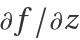, where z is complex. ResourceFunction["ComplexD"][f,Conjugate[z]] gives the partial derivative with respect to the complex conjugate of z. ResourceFunction["ComplexD"][f,{z,n}] gives the multiple derivative.

## Details and Options

Only derivatives of univariate functions are supported.
Complex derivatives are also known as Wirtinger derivatives.
Complex derivatives are defined by the equations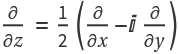and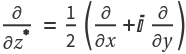, where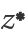is Conjugate[z]

## Examples

### Basic Examples (1)

Complex derivative of a function:

 In:=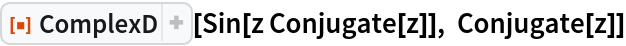Out=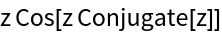### Scope (3)

ComplexD works with Abs:

 In:=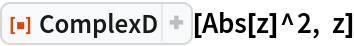Out=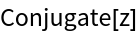Compare ComplexD with its definition for a function:

 In:=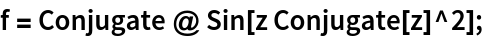Find the complex derivative using the definition in terms of derivatives with respect to the real and imaginary parts:

 In:=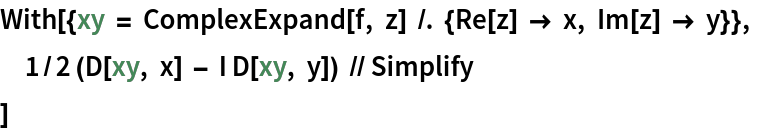Out=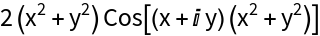Use ComplexExpand on the output of ComplexD:

 In:=Out=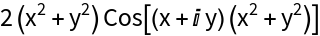More complicated expressions can be differentiated by first complex expanding the expression into one that consists of Conjugate only:

 In:=Out=### Applications (3)

Use ComplexD to find the real derivative (i.e. the derivative with respect to the real part only):

 In:=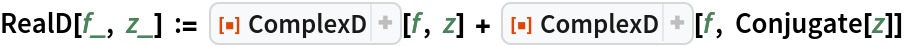For example, here is the "real" derivative of Abs:

 In:=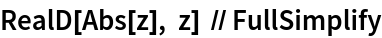Out=Compare to the usual definition of the real derivative (h is treated as real here):

 In:=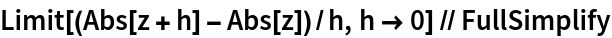Out=### Properties and Relations (1)

The complex variable z and its conjugate are independent:

 In:=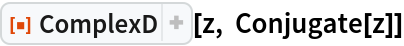Out=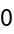In:=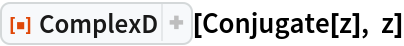Out=Carl Woll

## Version History

• 1.0.0 – 22 October 2019

## Author Notes

Support for multivariate functions could be added. Support for multiple derivatives could also be added (e.g., ComplexD[f, z, Conjugate[z]]).